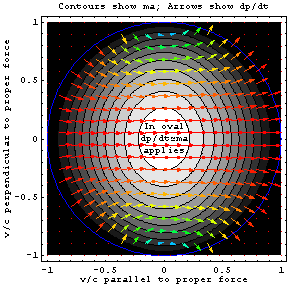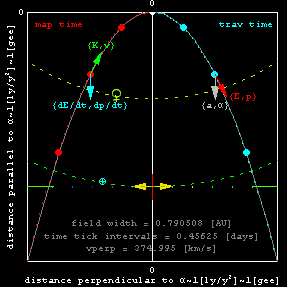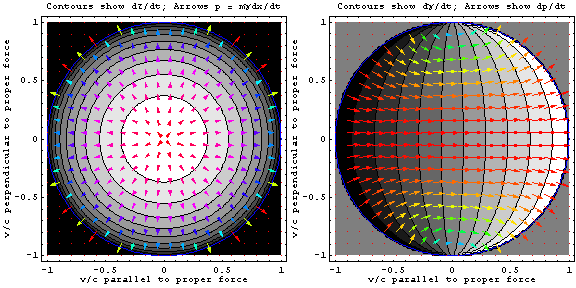Proper Force: When dp/dt ≠ ma."For Newton's Laws, relativity brought the bad news that coordinate-velocity has an upper limit and neither elapsed-time nor force are frame-invariant at high speed. The good news was that: (i) a 'one-gee round trip' takes travelers further than predicted for a given elapsed-time on their clocks, (ii) high speed colliders are a bargain because gamma-factors for relative proper-velocity multiply rather than add, (iii) acceleration properly-defined remains frame-invariant, and (iv) Newton's laws apply locally even in accelerated (non-rain) frames using geometric (connection-coefficient) forces like gravity, centrifugal, and Coriolis that act on every ounce of an object as if they might be removed by choice of a different frame.", Anonymous 2007

Proper-acceleration and proper-force are nothing more than the 3-vector acceleration and net force experienced in the frame of an accelerated object. Like proper-time and proper-length, they describe quantities from the vantage point of a special frame, in this case the frame of the object to which the acceleration and forces are applied. Their magnitude also happens to be the Lorentz-invariant magnitude of the acceleration and net force four-vectors seen from all frames, since in the rest frame of the accelerated object the time-like component of these four-vectors is zero. Because forces might be caused by objects moving with respect to one another, proper force Fo doesn't obey an action-reaction principle like 4-vector and locally-defined frame-variant forces do. However, it's an excellent bridge between locally-measured coordinate-acceleration a and force F=dp/dt (two things that Isaac Newton was fond of relating), since Fo is precisely maγ3 while F differs from Fo only when velocity is not-parallel to acceleration. In that case F's component parallel to Fo is divided by γ as F rotates toward the velocity line.

Using these observations, the figure at right illustrates relativistic adjustments to Newton's 2nd law (dp/dt~ma) over the domain of allowed values for coordinate-velocity dx/dt. For a fixed rightward proper-force Fo=mα and proper-acceleration α, the contours show how the magnitude of coordinate-acceleration a=d2x/dt2 (always parallel to proper-force) falls off as velocities approach lightspeed in any direction. In this way, coordinate-acceleration literally fades away as a useful concept at high speeds. The arrows, on the other hand, show how frame-variant force dp/dt falls off in magnitude as the component of coordinate-velocity perpendicular to proper-force increases, and how the direction of apparent force on an accelerated object aligns with its velocity direction, rather than with the proper-force that it experiences, as that velocity approaches c. Thus the white part of the bull's eye is the only region where relativistic adjustments to Newton's 2nd Law (dp/dt=ma) are small.

The equivalence principle of general relativity, combined with the concept of local affine-connection (or geometric) forces, makes proper-force useful even in non-inertial settings (like gravitationally-curved spacetime). The utility of Newton's Laws for describing gravity on earth as "just one more inter-object force" is living proof of that, since gravity (like magnetism) is an example of everyday relativity.

Below are some related plots. In the first plot, a trajectory for "1-gee" constant downward proper-acceleration is illustrated. This is the trajectory followed by a fly ball (neglecting air resistance) as well as by the Boeing 707 "vomit comet" used to give astronauts a taste of freefall, here implemented with help from a rocket engine on the accelerated object so that constancy can be maintained over much longer distances than possible with gravitational acceleration near the surface of a planet. Because the rocket trajectory is an accelerated trajectory in an unaccelerated frame (rather than a freefall trajectory in the presence of gravitational acceleration), the passengers on board will sense an earth-like gravity pulling down if their up is the direction of proper acceleration. As the field of view widens, you'll go from seeing only part of earth's orbit to seeing the sun and other planetary orbits, and eventually to seeing the Oort cloud and the nearby Centauri star-cluster shrink until the path looks like that of a Vogon demolition ship making a hyper-relativistic (127o) right turn. Is that cool or what?

Minimally-variant quantities like proper-time, relativistic energy and momentum (proper-velocity), and proper-acceleration are illustrated on the right-hand side of the plot while some highly frame-dependent but locally-useful counterparts (e.g. map-time, coordinate-velocity, kinetic-energy and rates of momentum/energy change) are shown on the left. The total duration of the "burn" on fixed-observer (map) clocks is eight times the listed interval between large dots on the trajectory profile (time ticks), and runs from 3.65 days to 100 years of earth time. This amounts to between 3.65 days and about 9 years on the clocks of those on board the spaceship. Imagine a drag racer capable of holding the pedal to the metal for days or years. We probably should have done experiments on this when gasoline was only 32¢ per gallon!The transverse velocity (vperp) is adjusted to keep the trajectory footprint square, the colored tick-marks have decimal separations of 10-6 through 100 lightyears, and the back to back arrows mark spatial separations increasing by powers of ten from 2×10-6 lightyears (yellow) to 2 lightyears (red). Notice the changes in shape from parabolic to hyperbolic as burn time increases. The transition occurs primarily over trajectories whose range is between 0.02 and 20 lightyears, as average velocity makes the transition from sub to hyper relativistic. Eventually the color of the arrows in this animation will convey information on the magnitude of the first (scalar) quantity labeling that arrow. The length of the arrow will convey information on the magnitude of the second (vector) quantity labeling that arrow. For the moment, only vector directions are conveyed. This changes most noticeably for the dp/dt vector on the left hand side, as relativistic Wigner rotation aligns the force seen in the map frame with the velocity line when the direction of proper acceleration remains fixed.

In the two plots on velocity space below, are those arrows perpendicular to those contours? If so, why?For those with a more technical bent, a set of fairly general equations for constant proper-acceleration α=Fo/m in the x-direction are listed by Mathematica below. Four-vectors are listed with the time-like component first. Using a Minkowski-signature of (+---), as expected u4·u4=c2, α4·α4=-α2, and u4·α4=0. One might generalize these further to arbitrary initial vx0/c = βx0, not by a Lorentz-boost in the acceleration direction (which with nonzero v Wigner-rotates the proper acceleration out of the observer's x-direction) but by replacing proper-time τ with τ - τo, where τo = -(c/α)ArcTanh[γβx0]. A bit of algebraic gymnastics with these equations might allow you, for example, to test the assertions here and/or to plot trajectories of your favorite projectile on the figures above.